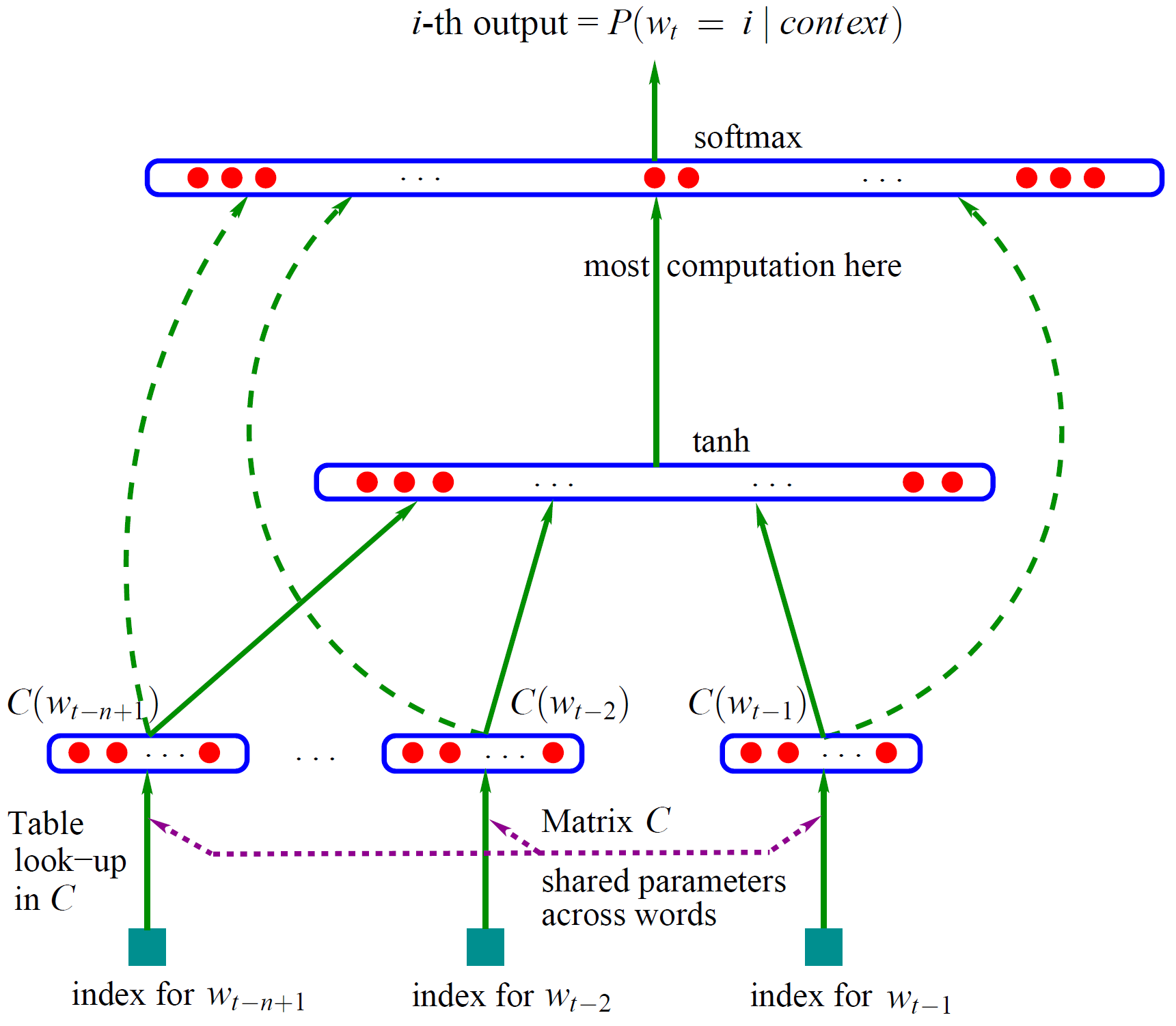Photo by### The model

1. the log-bilinear (LBL) model is perhaps the simplest neural language model.
2. Given the previous context words $$\{w_1,\cdots,w_{n-1}\}$$, the LBL model first predicts the representation for the next word $$w_n$$ by linearly combining the representations of the context words:
$\tilde r=\sum_{i=1}^{n-1}{C_i r_{w_i}}$

where, $$r_w$$ is the real-valued vector representing word $$w$$.

1. Then the distribution for the next word is computed based on the similarity between the predicted representation and the representations of all words in the vocabulary:
$P(w_n=w|w_{1:n-1})=\frac{\exp(\tilde r^\top r_w)}{\sum_j{\exp(\tilde r^\top r_j)}}$

### why it is called bilinear ?

• $$\tilde r$$ is the combination of the previous word embeddings
• $$r_w$$ is the embedding of word $w$.
• the multiplication of them $$\tilde r^\top r_w$$ is called bilinear map. As such, there only exists one embedding looking-up table.

### Bilinear map

In mathematics, a bilinear map is a function combining elements of two vector spaces to yield an element of a third vector space, and is linear in each of its arguments. Matrix multiplication is an example. A bilinear function (see https://en.wikipedia.org/wiki/Bilinear_map ) is a function which is linear in each variable when all other variables are fixed. For instance:

$f(x,y) = x * y$ $\log f(x,y)=\log x+\log y$